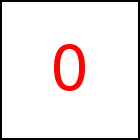# dV Library Method

Short Description: Set Depth Vector of Object

Signature: t.dV (x,y,z) or (p)
Group: Shape
Class: transition Class

Defines the depth vector of the animated object. Default is (0,0,0). For amimating 2D objects the depth vector is (almost) irrelevant, because 2D objects do not have a depth. More precisely for taccgl™ a 2D object is just the front face of a 3D box whereby all the other faces of the 3D box are invisible.

Sample HTML element with id="s10"

The depth vector gives the displacement from the top left front corner to the top left back corner. Alternatively (p) can be specified as a Position Specification that will be resolved into coordinates. p then specifies to top left back corner and the width vector is evaluated by subtracting the current top left front corner from p.

For 2D transitions just rotateMiddle takes the depth into account when calculating the middle to rotate around.

### Examples

 taccgl.actor("s10").rotateMiddle(1,0,0).start(); RUN taccgl.actor("s10").dV(0,0,500).rotateMiddle(1,0,0).start(); RUN

The following examples display a 3D box. By enlarging or reducing the vector, the depth of the animated object can be changed.

### Examples

 var a=taccgl.actor("s10",taccgl.dddBox) .start(); RUN var a=taccgl.actor("s10",taccgl.dddBox).dV(0,0,500).start(); RUN var a=taccgl.actor("s10",taccgl.dddBox).dV(200,0,500).start(); RUN var a=taccgl.actor("s10",taccgl.dddBox).dV(0,0,500).rotateMiddle(1,0,0).start(); RUN var a=taccgl.actor("s10",taccgl.dddBox).dV(0,0,50).rotateMiddle(1,0,0).start(); RUN

WebGL™ is a trademark of the Khronos Group Inc.

Next Page:transition.hVTo - Animate Height Vector of Object
Previous Page: transition.wV - Set Width Vector of Object

# Shape

taccgl™ - Canvas Drawing Library